# MOZ

The Ornstein-Zernike equations for mixtures (MOZ) of monomers with coincident oligomers (coincident dimers, trimers,...,n-mers).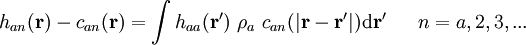$h_{an}({\mathbf r}) - c_{an}({\mathbf r}) = \int h_{aa} ({\mathbf r'})~\rho_a ~c_{an}(|{\mathbf r} - {\mathbf r'}|) {\rm d}{\mathbf r'}~~~~~n=a,2,3,...$

Since all oligomers are at infinite dilution, the OZ's for all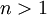$n>1$ are decoupled. The first member is for the bulk monomer fluid a (with size$\sigma$ and energy$\epsilon$)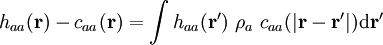$h_{aa}({\mathbf r}) - c_{aa}({\mathbf r}) = \int h_{aa} ({\mathbf r'})~\rho_a ~c_{aa}(|{\mathbf r} - {\mathbf r'}|) {\rm d}{\mathbf r'}$

For a coincident dimer (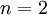$n=2$) of size$\sigma$ and energy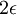$2\epsilon$ at infinite dilution in the bulk a-monomers: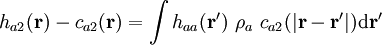$h_{a2}({\mathbf r}) - c_{a2}({\mathbf r}) = \int h_{aa} ({\mathbf r'})~\rho_a ~c_{a2}(|{\mathbf r} - {\mathbf r'}|) {\rm d}{\mathbf r'}$

For a coincident trimer (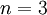$n=3$) of size$\sigma$ and energy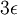$3\epsilon$ at infinite dilution in the bulk a-monomers: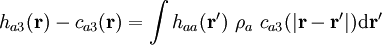$h_{a3}({\mathbf r}) - c_{a3}({\mathbf r}) = \int h_{aa} ({\mathbf r'})~\rho_a ~c_{a3}(|{\mathbf r} - {\mathbf r'}|) {\rm d}{\mathbf r'}$